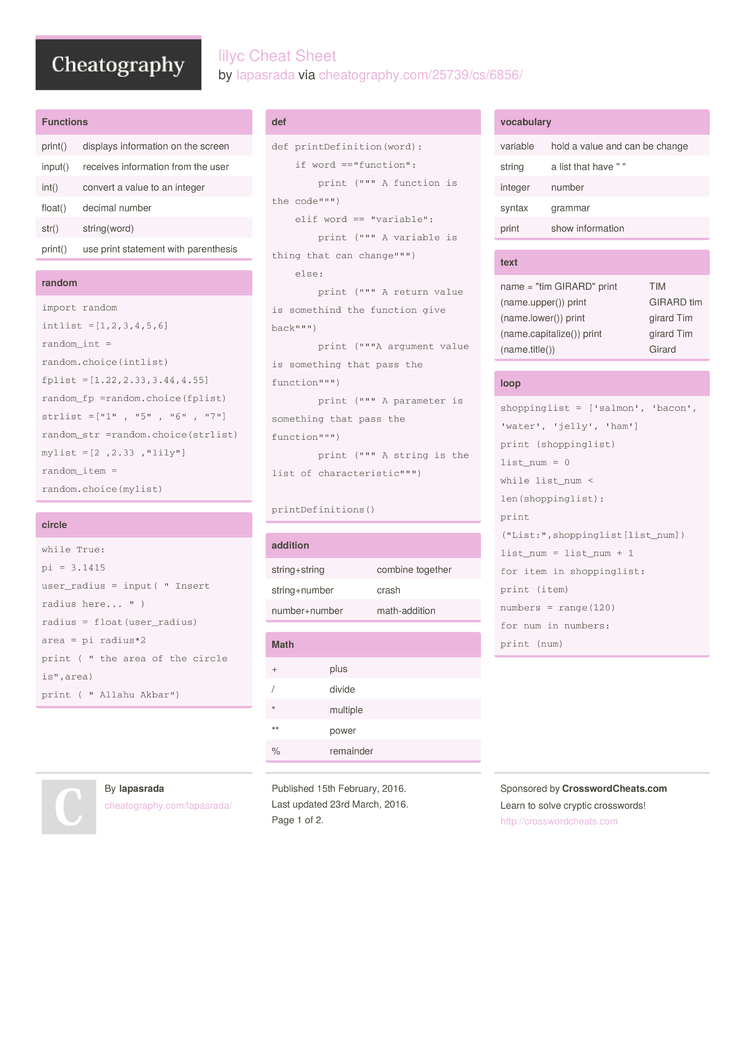Cheatography

# lilyc Cheat Sheet by lapasrada

### Functions

 print() displays inform­ation on the screen input() receives inform­ation from the user int() convert a value to an integer float() decimal number str() string­(word) print() use print statement with parent­hesis

### random

 ```import random intlist =[1,2,3,4,5,6] random_int = random.choice(intlist) fplist =[1.22,2.33,3.44,4.55] random_fp =random.choice(fplist) strlist =["1" , "5" , "6" , "7"] random_str =random.choice(strlist) mylist =[2 ,2.33 ,"lily"] random_item = random.choice(mylist)```

### circle

 ```while True: pi = 3.1415 user_r­adius = input( " Insert radius here... " ) radius = float(­use­r_r­adius) area = pi radius*2 print ( " the area of the circle is",­area) print ( " Allahu Akbar")```

### def

 ```def printDefinition(word):     if word =="function":         print (""" A function is the code""")     elif word == "variable":         print (""" A variable is thing that can change""")     else:         print (""" A return value is somethind the function give back""")         print ("""A argument value is something that pass the function""")         print (""" A parameter is something that pass the function""")         print (""" A string is the list of characteristic""")       printDefinitions()```

 string­+string combine together string­+number crash number­+number math-a­ddition

### Math

 + plus / divide * multiple ** power % remainder

### vocabulary

 variable hold a value and can be change string a list that have " " integer number syntax grammar print show inform­ation

### text

 name = "tim GIRARD­" print (name.u­pp­er()) print (name.l­ow­er()) print (name.c­ap­ita­lize()) print (name.t­it­le()) TIM GIRARD tim girard Tim girard Tim Girard

### loop

 ```shoppi­nglist = ['salmon', 'bacon', 'water', 'jelly', 'ham'] print (shopp­ing­list) list_num = 0 while list_num < len(sh­opp­ing­list): print ("Li­st:­"­,sh­opp­ing­lis­t[l­ist­_num]) list_num = list_num + 1 for item in shoppi­nglist: print (item) numbers = range(120) for num in numbers: print (num)```

### true false

 ```True or anything ==True False and anything ==false```

### if statment

 ```num= int(input(" enter a number")) if num<0:     print(num,"is negative") elif num==0:     print (num,"is zero") else:     print(num,"is possitive")```

### stop loop

 ```while True:     user_input=input("enter number:")     if user_input != "exit":         print(len(user_input))     else:         break```

### code

 ```while true:      user_radius = input(" what is the radius")      radius = float(user_radius)      pi = 3.1415      area = pi radius*2      print("the area of circle is", area)```

### code (copy)

 ```while true:      user_radius = input(" what is the radius")      radius = float(user_radius)      pi = 3.1415      area = pi radius*2      print("the area of circle is", area)```

### list

 ```import random intlist = [1, 2, 3, 4, 5, 6, 7, 8, 9, 10, 11, 12] random_int = random.choice (intlist) print(­int­lis­t,r­and­om_int) fplist = [0.1, 0.2, 0.3, 0.4, 0.5, 0.6] random_fp = random.choice (fplist) print (fplis­t,r­and­om_fp) strlist = ["1",­"­2","3­"­,"4",­"­5","6­"­,"7",­"­8","9­"] random_str = random.choice (strlist) print (strli­st,­ran­dom­_str) mylist = ["ad­am",­"­mil­d","l­ove­ada­m","l­evi­ne",­"­3","4.6",­424­,67­4,5.733] random­_item = random.choice (mylist) print (mylis­t,r­and­om_­item) myvar1 = 1 myvar2 = 2 myvar3 = 3 varlist = (myvar­1,m­yva­r2,­myvar3) random_var = random.choice (varlist) print (varli­st,­ran­dom­_var)```

### symbol

 ```if/eli­f/else condit­ionals While loop for list all the thing != If values of two operands are not equal, then condition becomes true. == test if the 2 value are the same < less than <= If the value of left operand is less than or equal to the value of right operand, then condition becomes true. > greater than >= If the value of left operand is greater than or equal to the value of right operand, then condition becomes true.```

### area

 ```def areaOfCirclr (r):     area = 3.14r*2     return area```

### function1

 ```def computeThis (a1,b2):     return a1*b2 a1 = int(input("Enter a number")) b2 = int(input("Enter a number")) print (computeThis (a1,b2))```2 Pages

PDF (recommended)